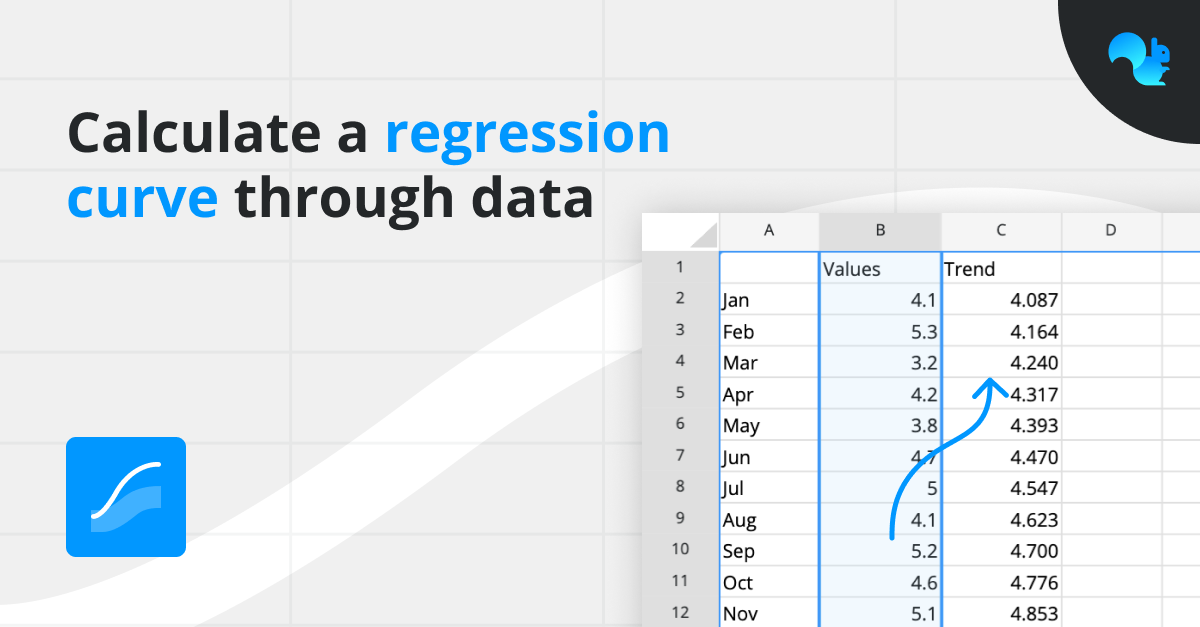# Trend

Developed by

### 0

Free## Calculate a trendline through a series of data

Calculates a regression trend line through a series of data
This Trend add-on will calculate a regression trend line through a series of datapoints.
Features

• Fit a linear, exponential, logarithmic, power or polynomial curve to the data
• Forecast points forward
• Output the R-squared statistic
• Get the equation of the fitted curve (both as text and as numeric coefficients)